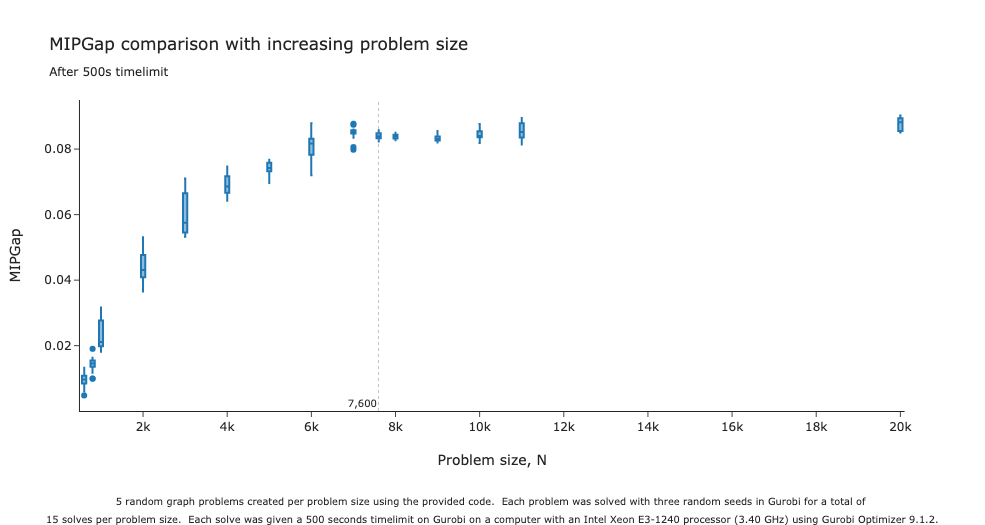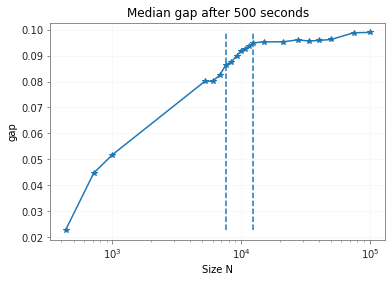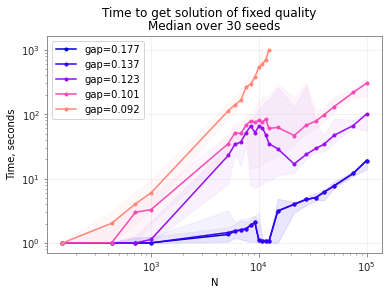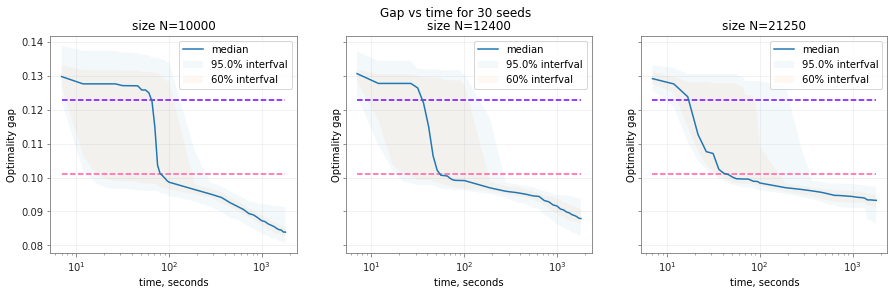•Gurobi Staff

Thanks for the nice code example and graphs. Yes, problem size impacts a lot of different decisions Gurobi makes.

Is there a reason you are running your tests with Threads=1? The extra threads may help a lot on these models, especially for finding new incumbent solutions at the root node. I expect using the default value of the Threads parameter would resolve some of the performance difference you are seeing.

Additionally, you could try the following parameters:

• Symmetry=0 disables symmetry detection. In the log file for the N=7600 model, Gurobi spends the entire solve (100 seconds) at the root node. This time may be spent looking for symmetries in the model, which could be particularly damaging to performance when limiting Gurobi to a single thread.
• PreQLinearize controls how/if Gurobi linearizes the quadratic objective. Gurobi opts for a "strong" reformulation on both of these models (PreQLinearize=1). Surprisingly, PreQLinearize=0 seems promising in some quick tests I ran using your code. You could also try PreQLinearize=2, which tells Gurobi to build a compact reformulation of the quadratic objective.
•I run gurobi mostly to time its performance for different-sized problems. I solve MaxCut on a multitude of different graphs and parallelize over them, such that each thread on my server runs optimization for a single graph. Let's say I have 50 cores and start to optimize 50 graphs in parallel. If gurobi itself runs in parallel, it will over-load the cores and increase timings. However, I might run 25 graphs in parallel and give gurobi 2 threads.
In general, I don't want the parallelization of gurobi to influence the timings for each individual problem.

I'll try the changing the parameters you mentioned and will get back to you with results!

•Gurobi Staff

Eli's suggestions are the right way to go to improve performance overall.  But, out of curiosity, I did some additional digging using the code you provided to better understand the performance profile with respect to problem size.

I found that if you expand the range of problem sizes tested, performance decreases with increasing problem size as you expected.  However, the performance decreases seem to level off when the problem size, N, exceeds five or six thousand.  You can see this in the box plot provided below.•Hi Alison! Thank you for the plot.

I run Gurobi with these parameters: {'Symmetry': 0, 'PreQLinearize': 2} and found that for them the saturation point is a bit different.The plot was done using 30 random 3-regular graphs. The first vertical line is N=7600, the second is N=12400.
I chose PreQLinearize=2 because for PreQLinearize=0 gurobi was spending a lot of time in some intermediary state before starting to explore MIP nodes. For Symmetry=0,  PreQLinearize=2 the solution is a bit better, but the strange behavior is still there, albeit for different N.

Here's a plot of time to reach a particular gap vs N for different gaps.You can see that it's easier to get a gap of 0.123 for N~20k  than for N=10k. Here's the analogous plot for gap vs time for the sizes in question:The horizontal lines correspond to gap=0.123 and 0.101 to reference with the previous plot.

What is also interesting is that all of the sizes have this sharp drop in gap in the gap vs time plot, and then a gradual descent. It's the time at which this drop occurs that becomes smaller vs N for N~10k-20k